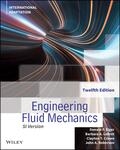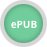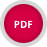John Wiley & Sons Engineering Fluid MechanicsEngineering Fluid Mechanics 12th edition guides students from theory to application, emphasizing ski.. Product #: 978-1-119-82073-4 Regular price: \$55.98 \$55.98 Auf Lager

# Engineering Fluid Mechanics12. Auflage April 2022
608 Seiten, Softcover
Lehrbuch

ISBN: 978-1-119-82073-4
John Wiley & Sons

Jetzt kaufen

### Preis: 59,90 €

Preis inkl. MwSt, zzgl. Versand

Weitere VersionenEngineering Fluid Mechanics 12th edition guides students from theory to application, emphasizing skills like critical thinking, problem solving and modeling to apply fluid mechanics concepts to solve real-world engineering problems. The essential concepts are presented in a clear and concise format, while abundant illustrations, charts, diagrams, and examples illustrate complex topics and highlight the physical reality of fluid dynamics applications. The text emphasizes on technical derivations, presenting derivations of main equation in a step-by-step manner and explaining their holistic meaning in words. The Wales-Wood Model is used throughout the text to solve numerous example problems. This International Adaptation comes with some updates that enhance and expand certain concepts and some organizational changes. The edition provides a wide variety of new and updated solved problems, real-world engineering examples, and end-of-chapter homework problems and has been completely updated to use SI units.The text, though written from civil engineering perspective, adopts an interdisciplinary approach which makes it suitable for engineering students of all majors who are taking a first or second course in fluid mechanics.

1. Introduction

1.1 Engineering Fluid Mechanics

1.2 Modeling in Fluid Mechanics and Engineering

1.3 Modeling of Materials

1.4 Weight, Mass, and Newton's Law of Gravitation

1.5 Essential Mathematics Topics

1.6 Density and Specific Weight

1.7 The Ideal Gas Law (IGL)

1.8 Quantity, Units, and Dimensions

1.9 Problem Solving

1.10 Summarizing Key Knowledge

Problems

2. Fluid Properties

2.1 System, State, and Property

2.2 Looking Up Fluid Properties

2.3 Specific Gravity, Constant Density, and the Bulk Modulus

2.4 Pressure and Shear Stress

2.5 The Viscosity Equation

2.6 Surface Tension and Capillary Action

2.7 Vapor Pressure, Boiling, and Cavitation

2.8 Characterizing Thermal Energy in Flowing Gases

2.9 Summarizing Key Knowledge

Problems

3. Fluid Statics

3.1 Describing Pressure

3.2 The Hydrostatic Equations

3.3 Measurement of Pressure

3.4 The Pressure Force on a Panel (Flat Surface)

3.5 Calculating the Pressure Force on a Curved Surface

3.6 Calculating Buoyant Forces

3.7 Predicting Stability of Immersed and Floating Bodies

3.8 Summarizing Key Knowledge

Problems

4. Bernoulli Equation and Pressure Variation

4.1 Flow Patterns: Streamlines, Streaklines, and Pathlines

4.2 Characterizing Velocity of a Flowing Fluid

4.3 Describing Flow

4.4 Acceleration

4.5 Applying Euler's Equation to Understand Pressure Variation

4.6 The Bernoulli Equation along a Streamline

4.7 Measuring Velocity and Pressure

4.8 Characterizing the Rotational Motion of a Flowing Fluid

4.9 The Bernoulli Equation for Irrotational Flow

4.10 Describing the Pressure Field for Flow over a Circular Cylinder

4.10 Elementary Plane potential Flows

4.11 Calculating the Pressure Field for a Rotating Flow

4.12 Summarizing Key Knowledge

Problems

5. The Control Volume Approach and The Continuity Equation

5.1 Characterizing the Rate of Flow

5.2 The Control Volume Approach

5.3 The Continuity Equation (Theory)

5.4 The Continuity Equation (Application)

5.5 Predicting Cavitation

5.6 Summarizing Key Knowledge

6. The Momentum Equation

6.1 Understanding Newton's Second Law of Motion

6.2 The Linear Momentum Equation: Theory

6.3 The Linear Momentum Equation: Application

6.4 The Linear Momentum Equation for a Stationary Control Volume

6.5 Examples of the Linear Momentum Equation (Moving Objects)

6.6 The Angular Momentum Equation

6.7 Summarizing Key Knowledge

Problems

7. The Energy Equation

7.1 Technical Vocabulary: Work, Energy, and Power

7.2 Conservation of Energy

7.3 The Energy Equation

7.4 The Power Equation

7.5 Mechanical Efficiency

7.6 Contrasting the Bernoulli Equation and the Energy Equation

7.7 Transitions

7.8 The Hydraulic and Energy Grade Lines

7.9 Summarizing Key Knowledge

Problems

8. Dimensional Analysis and Similitude

8.1 The Need for Dimensional Analysis

8.2 Buckingham n-ary product Theorem

8.3 Dimensional Analysis

8.4 Common pi-Groups

8.5 Similitude

8.6 Model Studies for Flows without Free-Surface Effects

8.7 Model-Prototype Performance

8.8 Approximate Similitude at High Reynolds Numbers

8.9 Free-Surface Model Studies

8.10 Summarizing Key Knowledge

Problems

9. Viscous Flow Over a Flat Surface, Drag and Lift

9.1 The Navier-Stokes Equation for Uniform Flow

9.2 Couette Flow

9.3 Poiseuille Flow in a Channel

9.4 The Boundary Layer (Description)

9.5 Velocity Profiles in the Boundary Layer

9.6 The Boundary Layer (Calculations)

9.7 Relating Lift and Drag to Stress Distributions

9.8 Calculating the Drag Force

9.9 Drag of Axisymmetric and 3-D Bodies

9.10 Terminal Velocity

9.11 Vortex Shedding

9.12 Reducing Drag by Streamlining

9.13 Drag in Compressible Flow

9.14 The Theory of Lift

9.15 Lift and Drag on Airfoils

9.16 Lift and Drag on Road Vehicles

9.17 Summarizing Key Knowledge

Problems

10. Flow in Conduits

10.1 Classifying Flow

10.2 Specifying Pipe Sizes

10.3 Pipe Head Loss (Major and Minor losses)

10.4 Stress Distributions in Pipe Flow

10.5 Laminar Flow in a Circular Pipe

10.6 Turbulent Flow and the Moody Chart

10.7 A Strategy for Solving Problems

10.9 Noncircular Conduits

10.10 Pumps and Systems of Pipes

10.11 Summarizing Key Knowledge

Problems

11. Compressible Flow

11.1 Wave Propagation in Compressible Fluids

11.2 Mach Number Relationships

11.3 Normal Shock Waves

11.4 Isentropic Compressible Flow through a Duct with Varying Area

11.5 Summarizing Key Knowledge

Problems

12. Flow Measurements

12.1 Measuring Velocity and Pressure

12.2 Measuring Flow Rate (Discharge)

12.3 Summarizing Key Knowledge

Problems

13.Turbomachinery

13.1 Propellers

13.2 Axial-Flow Pumps

13.4 Specific Speed

13.5 Suction Limitations of Pumps

13.6 Viscous Effects

13.7 Centrifugal Compressors

13.8 Positive Displacement Pumps

13.9 Turbines

13.10 Summarizing Key Knowledge

Problems

14. Flow in Open Channels

14.1 Describing Open-Channel Flow

14.2 Energy Equation for Steady Open-Channel Flow

14.5 Rapidly Varied Flow

14.6 Hydraulic Jump

14.8 Summarizing Key Knowledge

Problems

15. Modeling of Fluid Dynamics Problems

15.1 Models in Fluid Mechanics

15.2 Foundations for Learning Partial Differential Equations (PDEs)

15.3 The Continuity Equation

15.4 The Navier-Stokes Equation

15.5 Computational Fluid Dynamics (CFD)

15.6 Examples of CFD

15.7 A Path for Moving Forward

15.8 Summarizing Key Knowledge

Problems

Appendix